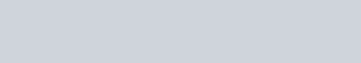An element X (atomic number 17) reacts
Question:

An element X (atomic number 17) reacts with an element Y (atomic number 20) to form a divalent halide.

(a) Where in the Periodic Table are elements X and Y placed ?

(b) Classify X and Y as metal(s), non-metal(s) or metalloid(s).

(c) What will be the nature of oxide of element Y ? Identify the nature of bonding in the compound formed

(d) Draw the electron dot structure of the divalent halide.

Solution:

Element X (Z = 17) is chlorine while element Y (Z = 20) is calcium.

(a) Chlorine (Cl) is a member of group 17 and period 3. Calcium (Ca) is present in group 2 and period 4.

(b) Chlorine is a typical non-metal while calcium is a metal.

(c) The oxide of calcium is calcium oxide (CaO). It is a basic oxide.